# Solving Exponential Equations with Logarithms

Exponential equations can be solved by taking the log of both sides.

Steps:

1) As much as possible, get the log by itself.

2) Take the log (or natural log) of both sides.

3) Simplify as needed using the log rules.

4) Solve the equation for the variable.

Examples:

1.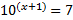The log is by itself, so we will take the log of both sides: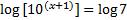We can use the log rules to simplify the left hand side: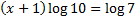Since log 10 = 1, we now have....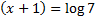Note: Notice that the log and the 10 cancel and the exponent is left by itself. You can go through the process above each time or you can remember this pattern.

Let's finish solving this equation: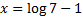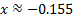2.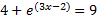First let's get the natural log by itself by moving the 4: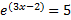Now we'll take the natural log of both sides: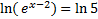We can use the log rules to simplify the left hand side: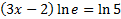Since ln e = 1, we now have....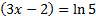Or, we could've simply remembered that the ln and e cancel, leaving the exponent by itself.

Let's finish solving this equation: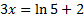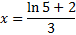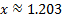3.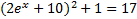First we must get the e by itself. Weâll move the 1 first: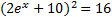Then take the square root of both sides: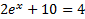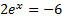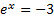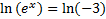At this point we realize we have a problem. We cannot take ln (-3) because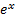cannot equal a negative number. Thus, there is no solution to this equation.

Practice: Solve each exponential equation. If necessary, round your answer to the nearest thousandth.

1)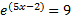2)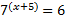3)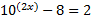4)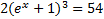5)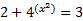Answers: 1)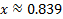2)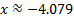3)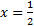4)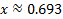5) x = 0

 Related Links: Math Fractions Factors